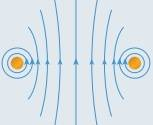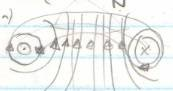# Where is North, given this current?

• AAAA
In summary, both Figures 1 and 2 produce the same magnetic field lines, but the direction of the field lines changes depending on which direction the current is coming from. North is up in Figure 2 because the current is coming from the top.

## Homework Statement

Copy the diagrams in Figure 7 into your notebook, and draw the direction of the conventional current or the
magnetic field lines. Also indicate the location of the north and south poles where appropriate.

FIGURE 1FIGURE 2## The Attempt at a Solution

Using conventional flow (+ve →-ve)

Firstly, would I be correct in assuming that both Figure 1 and Figure 2 would produce the same magnetic field lines? Because using the right hand rule (which my book uses), a current going out of the page (left circle, figure 1) would produce field lines going counter-clockwise, and into the page (right circle, figure 1) is clockwise using the right hand rule. So would the two be the exact same?

What I can't seem to figure out is which way is north. I know how to determine north in a solenoid, but I'm not sure how to determine it for just two currents. Is there a north/south? We weren't taught this in class, and it doesn't seem to be in my textbook.

Thanks for any help.

Last edited:
In your figure 1, the circle on the left with the dot in its center represents a current (or field, or vector) coming OUT of the page. The other circle, with the cross, represents something going INTO the page. The imagery harkens back to a vector being represented by an arrow. The tip is a point, the tail is the fletching (feathers in an X shape). So an arrow just coming through a surface in your direction would be seen as just the point of the arrow head. An arrow just disappearing into the surface would present just the fletching of the tail.

So, given the above correction for the directions, what's your evaluation of the magnetic field line directions?

Sorry, my mistake, I typed it wrong, it's fixed now.

The directions would be what I said earlier, no? On the right it's clock wise, the left is counter-clockwise? So the figure 1 is the same as figure 2 then? I'm still not sure about where north would be.

As for the poles... How would you solve it if the diagram represented a cross section through a one turn solenoid?

•AAAA
Right, I did it like that at one point, when I tried to solve it:That's how I deduced it before , so that north is up. I just didn't put it up because I had a previous thread here that was taken down in part because I didn't show any effort to solve the problem. So I thought it was incorrect.

AAAA said:
Right, I did it like that at one point, when I tried to solve it:That's how I deduced it before , so that north is up. I just didn't put it up because I had a previous thread here that was taken down in part because I didn't show any effort to solve the problem. So I thought it was incorrect.

Lack of effort is not quite the same thing as being incorrect :)

Okay :)
So North is up in this diagram (Figure 2), and because they're the same→ Figure 1 as well?

AAAA said:
Okay :)
So North is up in this diagram (Figure 2), and because they're the same→ Figure 1 as well?
Don't be afraid to make a statement rather than a question :). If the field lines are the same for both cases then you should be able to draw the conclusion with certainty.

That said, yes, they both represent the same scenario, and north is at the top as you've drawn it.

•AAAA
Alright. Thanks to you both!
gneill said:
Don't be afraid to make a statement rather than a question
That'll ↑ be going up on my bedroom wall :D

Why is up north, for this question? I know I can explain using that solenoid. But what about the reasoning, at a high level. I know that field lines go from north to south, but why?

AAAA said:
Why is up north, for this question? I know I can explain using that solenoid. But what about the reasoning, at a high level. I know that field lines go from north to south, but why?
Take a look at the field lines for a solenoid and bar magnet. Note the circulation pattern and especially the direction of the field line arrows internal to the magnet versus outside the magnet.

•AAAA
AAAA said:
Why is up north, for this question? I know I can explain using that solenoid.

Why is it any different?

•AAAA
Okay, thanks. Everything makes sense now